Next: Cluster cardinality in K-means Up: Flat clustering Previous: Evaluation of clustering   Contents   Index

# K-means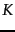-means is the most important flat clustering algorithm. Its objective is to minimize the average squared Euclidean distance (Chapter 6 , page 6.4.4 ) of documents from their cluster centers where a cluster center is defined as the mean or centroid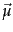of the documents in a cluster: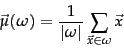(190)

The definition assumes that documents are represented as length-normalized vectors in a real-valued space in the familiar way. We used centroids for Rocchio classification in Chapter 14 (page 14.2 ). They play a similar role here. The ideal cluster in-means is a sphere with the centroid as its center of gravity. Ideally, the clusters should not overlap. Our desiderata for classes in Rocchio classification were the same. The difference is that we have no labeled training set in clustering for which we know which documents should be in the same cluster.

A measure of how well the centroids represent the members of their clusters is the residual sum of squares or RSS , the squared distance of each vector from its centroid summed over all vectors: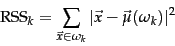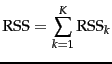(191)

RSS is the objective function in-means and our goal is to minimize it. Since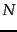is fixed, minimizing RSS is equivalent to minimizing the average squared distance, a measure of how well centroids represent their documents.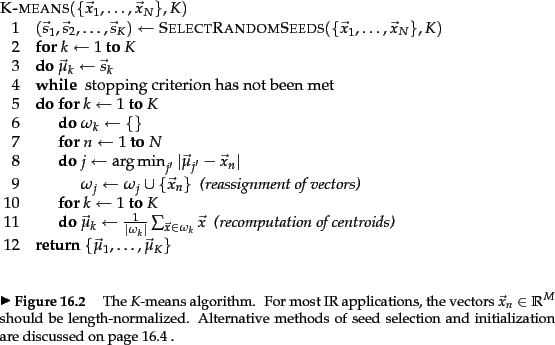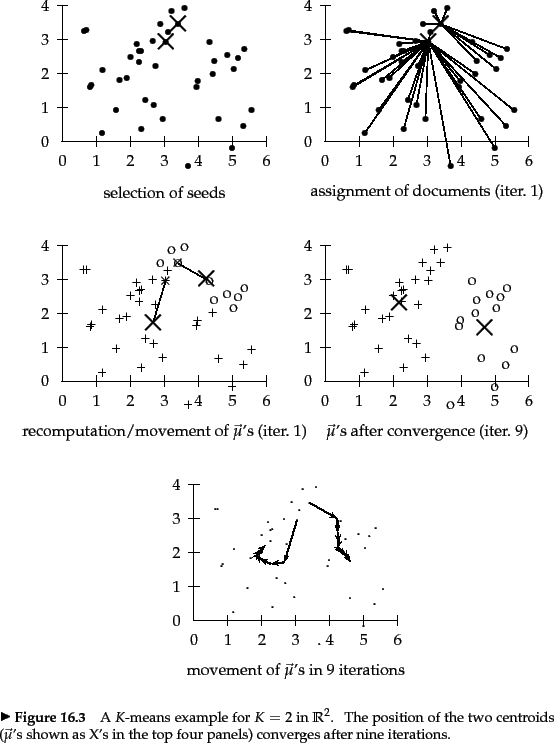The first step of-means is to select as initial cluster centersrandomly selected documents, the seeds . The algorithm then moves the cluster centers around in space in order to minimize RSS. As shown in Figure 16.5 , this is done iteratively by repeating two steps until a stopping criterion is met: reassigning documents to the cluster with the closest centroid; and recomputing each centroid based on the current members of its cluster. Figure 16.6 shows snapshots from nine iterations of the-means algorithm for a set of points. The centroid'' column of Table 17.2 (page 17.2 ) shows examples of centroids.

We can apply one of the following termination conditions.

• A fixed number of iterations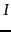has been completed. This condition limits the runtime of the clustering algorithm, but in some cases the quality of the clustering will be poor because of an insufficient number of iterations.
• Assignment of documents to clusters (the partitioning function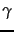) does not change between iterations. Except for cases with a bad local minimum, this produces a good clustering, but runtimes may be unacceptably long.
• Centroids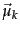do not change between iterations. This is equivalent tonot changing (Exercise 16.4.1 ).
• Terminate when RSS falls below a threshold. This criterion ensures that the clustering is of a desired quality after termination. In practice, we need to combine it with a bound on the number of iterations to guarantee termination.
• Terminate when the decrease in RSS falls below a threshold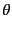. For small, this indicates that we are close to convergence. Again, we need to combine it with a bound on the number of iterations to prevent very long runtimes.

We now show that-means converges by proving that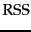monotonically decreases in each iteration. We will use decrease in the meaning decrease or does not change in this section. First, RSS decreases in the reassignment step since each vector is assigned to the closest centroid, so the distance it contributes todecreases. Second, it decreases in the recomputation step because the new centroid is the vector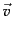for which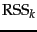reaches its minimum.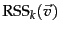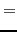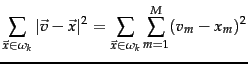(192)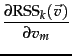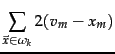(193)

where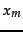and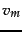are the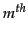components of their respective vectors. Setting the partial derivative to zero, we get: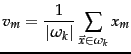(194)

which is the componentwise definition of the centroid. Thus, we minimizewhen the old centroid is replaced with the new centroid., the sum of the, must then also decrease during recomputation.

Since there is only a finite set of possible clusterings, a monotonically decreasing algorithm will eventually arrive at a (local) minimum. Take care, however, to break ties consistently, e.g., by assigning a document to the cluster with the lowest index if there are several equidistant centroids. Otherwise, the algorithm can cycle forever in a loop of clusterings that have the same cost.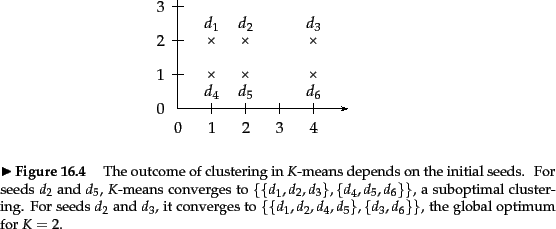While this proves the convergence of-means, there is unfortunately no guarantee that a global minimum in the objective function will be reached. This is a particular problem if a document set contains many outliers , documents that are far from any other documents and therefore do not fit well into any cluster. Frequently, if an outlier is chosen as an initial seed, then no other vector is assigned to it during subsequent iterations. Thus, we end up with a singleton cluster (a cluster with only one document) even though there is probably a clustering with lower RSS. Figure 16.7 shows an example of a suboptimal clustering resulting from a bad choice of initial seeds.

Another type of suboptimal clustering that frequently occurs is one with empty clusters (Exercise 16.7 ).

Effective heuristics for seed selection include (i) excluding outliers from the seed set; (ii) trying out multiple starting points and choosing the clustering with lowest cost; and (iii) obtaining seeds from another method such as hierarchical clustering. Since deterministic hierarchical clustering methods are more predictable than-means, a hierarchical clustering of a small random sample of size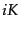(e.g., for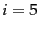or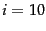) often provides good seeds (see the description of the Buckshot algorithm, Chapter 17 , page 17.8 ).

Other initialization methods compute seeds that are not selected from the vectors to be clustered. A robust method that works well for a large variety of document distributions is to select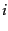(e.g.,) random vectors for each cluster and use their centroid as the seed for this cluster. See Section 16.6 for more sophisticated initializations.

What is the time complexity of-means? Most of the time is spent on computing vector distances. One such operation costs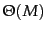. The reassignment step computes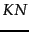distances, so its overall complexity is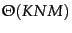. In the recomputation step, each vector gets added to a centroid once, so the complexity of this step is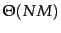. For a fixed number of iterations, the overall complexity is therefore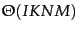. Thus,-means is linear in all relevant factors: iterations, number of clusters, number of vectors and dimensionality of the space. This means that-means is more efficient than the hierarchical algorithms in Chapter 17 . We had to fix the number of iterations, which can be tricky in practice. But in most cases,-means quickly reaches either complete convergence or a clustering that is close to convergence. In the latter case, a few documents would switch membership if further iterations were computed, but this has a small effect on the overall quality of the clustering.

There is one subtlety in the preceding argument. Even a linear algorithm can be quite slow if one of the arguments of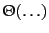is large, and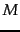usually is large. High dimensionality is not a problem for computing the distance between two documents. Their vectors are sparse, so that only a small fraction of the theoretically possiblecomponentwise differences need to be computed. Centroids, however, are dense since they pool all terms that occur in any of the documents of their clusters. As a result, distance computations are time consuming in a naive implementation of-means. However, there are simple and effective heuristics for making centroid-document similarities as fast to compute as document-document similarities. Truncating centroids to the most significant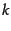terms (e.g.,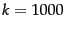) hardly decreases cluster quality while achieving a significant speedup of the reassignment step (see references in Section 16.6 ).

The same efficiency problem is addressed by K-medoids , a variant of-means that computes medoids instead of centroids as cluster centers. We define the medoid of a cluster as the document vector that is closest to the centroid. Since medoids are sparse document vectors, distance computations are fast.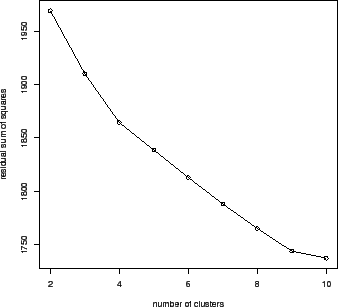Estimated minimal residual sum of squares as a function of the number of clusters in-means. In this clustering of 1203 Reuters-RCV1 documents, there are two points where the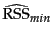curve flattens: at 4 clusters and at 9 clusters. The documents were selected from the categories China, Germany, Russia and Sports, so the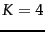clustering is closest to the Reuters classification.

SubsectionsNext: Cluster cardinality in K-means Up: Flat clustering Previous: Evaluation of clustering   Contents   Index